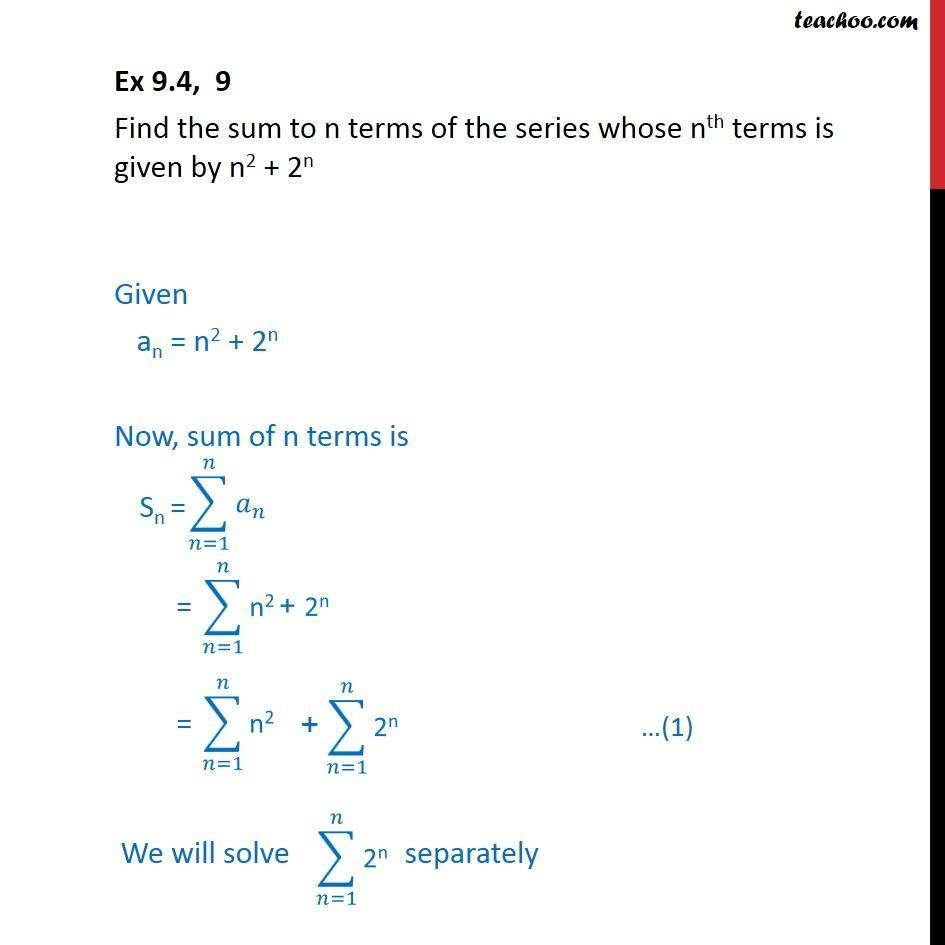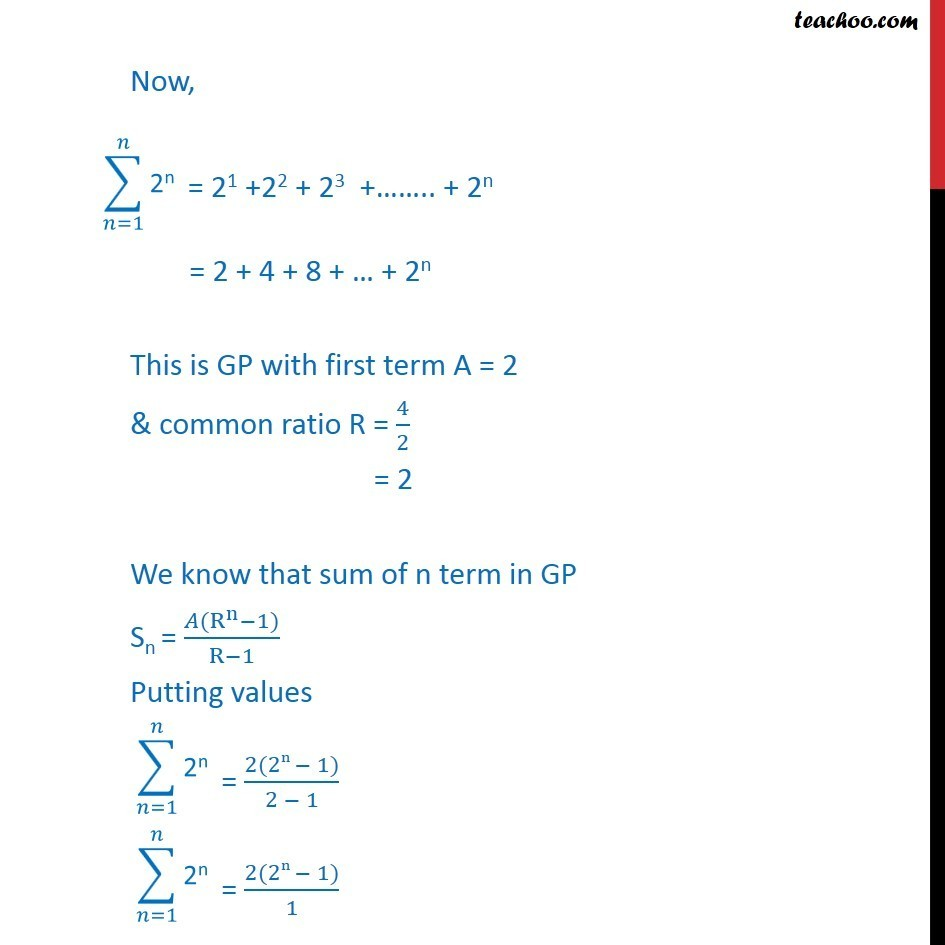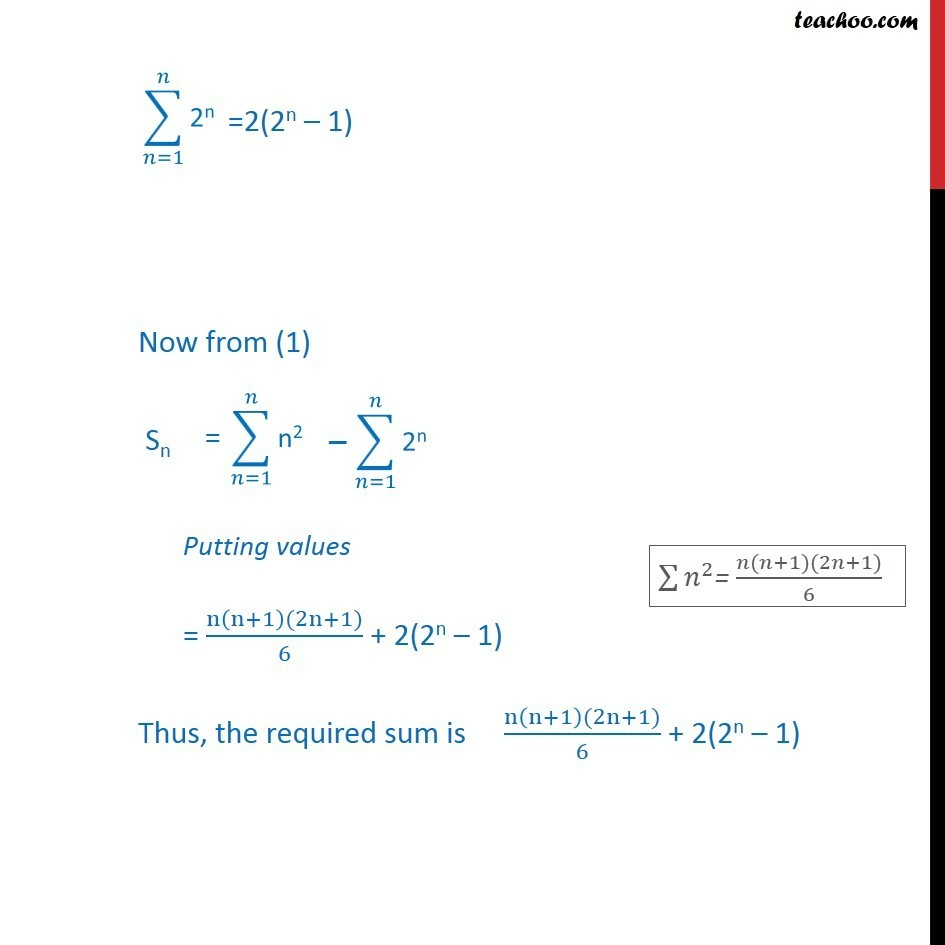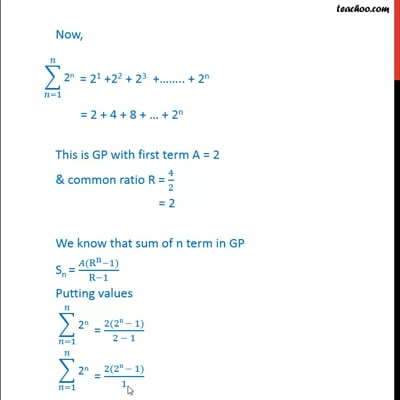Ex 9.4

Chapter 9 Class 11 Sequences and Series
Serial order wiseThis video is only available for Teachoo black users

Get live Maths 1-on-1 Classs - Class 6 to 12

### Transcript

Ex 9.4, 9 Find the sum to n terms of the series whose nth terms is given by n2 + 2n Given an = n2 + 2n Now, sum of n terms is Now, = 2 + 4 + 8 + + 2n This is GP with first term A = 2 & common ratio R = 4/2 = 2 We know that sum of n term in GP Sn = ( (R^n 1))/(R 1) Putting values Now from (1) Putting values = (n(n+1)(2n+1))/6 + 2(2n 1) Thus, the required sum is (n(n+1)(2n+1))/6 + 2(2n 1)